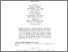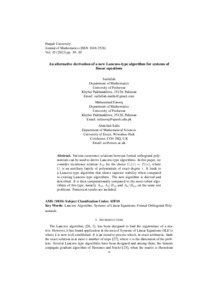# An alternative derivation of a new Lanczos-type algorithm for systems of linear equations

Ullah, Saif and Farooq, Muhammad and Salhi, Abdellah An alternative derivation of a new Lanczos-type algorithm for systems of linear equations. UNSPECIFIED. UNSPECIFIED.Preview
Text
paper5_v45_2013.pdf

Download (105kB) | Preview

## Abstract

Various recurrence relations between formal orthogonal polynomials can be used to derive Lanczos-type algorithms. In this paper, we consider recurrence relation \$A_{12}\$ for the choice \$U_i(x)=P_i(x)\$, where \$U_i\$ is an auxiliary family of polynomials of exact degree \$i\$. It leads to a Lanczos-type algorithm that shows superior stability when compared to existing Lanczos-type algorithms. The new algorithm is derived and described. It is then computationally compared to the most robust algorithms of this type, namely \$A_{12}\$, \$A_5/B_{10}\$ and \$A_8/B_{10}\$, on the same test problems. Numerical results are included.

Item Type: Monograph (UNSPECIFIED) math.NA, math.NA Q Science > QA Mathematics Faculty of Science and Health > Mathematical Sciences, Department of Jim Jamieson 12 Nov 2014 14:27 04 Jul 2018 13:15 http://repository.essex.ac.uk/id/eprint/11538

### Actions (login required)View Item